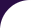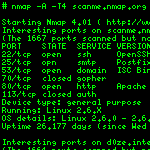Intro Reference Guide Book Install Guide Download Changelog Zenmap GUI Docs Bug Reports OS Detection Propaganda Related Projects In the Movies In the News# Library `formulas`

Formula functions for various calculations.

The library lets scripts to use common mathematical functions to compute percentages, averages, entropy, randomness and other calculations. Scripts that generate statistics and metrics can also make use of this library.

## Functions

 calcPwdEntropy (value) Calculate the entropy of a password. looksRandom (data) Checks whether a sample looks random mean_stddev (t) Return the mean and sample standard deviation of an array, using the algorithm from Knuth Vol. 2, Section 4.2.2. median (t) Find the median of a list quickselect (t, k) Return the k-th largest element in a list

## Functions

calcPwdEntropy (value)

Calculate the entropy of a password.

A random password's information entropy, H, is given by the formula: H = L * (logN) / (log2), where N is the number of possible symbols and L is the number of symbols in the password. Based on https://en.wikipedia.org/wiki/Password_strength

### Parameters

• value: The password to check

### Return value:

The entropy in bits
looksRandom (data)

Checks whether a sample looks random

Because our sample is so small (only 16 bytes), do a chi-square goodness of fit test across groups of 2, 4, and 8 bits. If using only 8 bits, for example, any sample whose bytes are all different would pass the test. Using 2 bits will tend to catch things like pure ASCII, where one out of every four samples never has its high bit set.

### Parameters

• data: The data to check

### Return value:

True if the data appears to be random, false otherwise
mean_stddev (t)

Return the mean and sample standard deviation of an array, using the algorithm from Knuth Vol. 2, Section 4.2.2.

• t:

### Return values:

1. The mean of the values
2. The standard deviation of the values
median (t)

Find the median of a list

### Parameters

• t: the table/list of values

### Return value:

the median value
quickselect (t, k)

Return the k-th largest element in a list

### Parameters

• t: The list, not sorted
• k: The ordinal value to return

### Return value:

The k-th largest element in the list